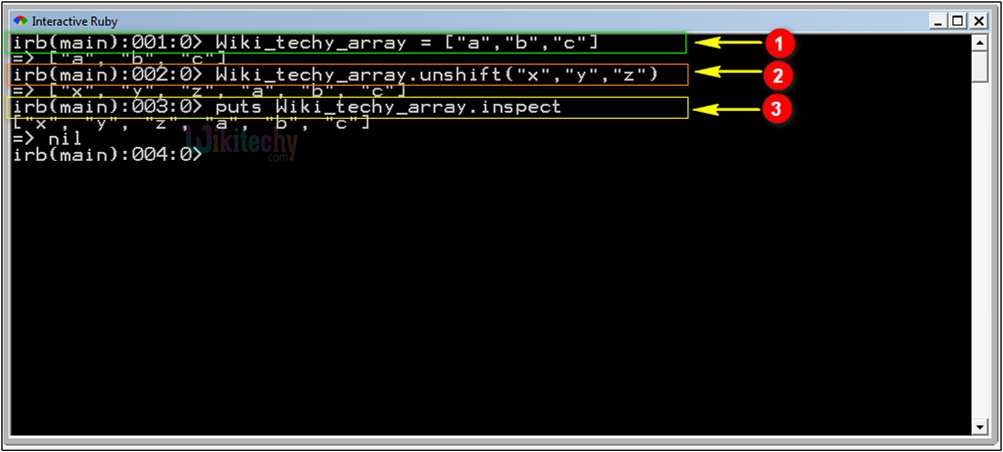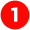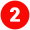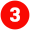# Ruby - Array UnShift

## Sample ruby code :

• unshift appends elements to the beginning of an array.
```Wiki_techy_array = ["a","b","c"]
Wiki_techy_array.unshift("x","y","z")
puts Wiki_techy_array.inspect```

## Code Explanation :An array variable Wiki_techy_array is declared with values a, b, c.The code Wiki_techy_array.unshift("x","y","z") shifts in the values x, y, z into the array’s initial point. Now the array values are x, y, z, a, b, c.The line of code puts Wiki_techy_array.inspect prints the array values as follows: ["x", "y", "z", "a", "b", "c"]

IOT Hackathon
Webinar